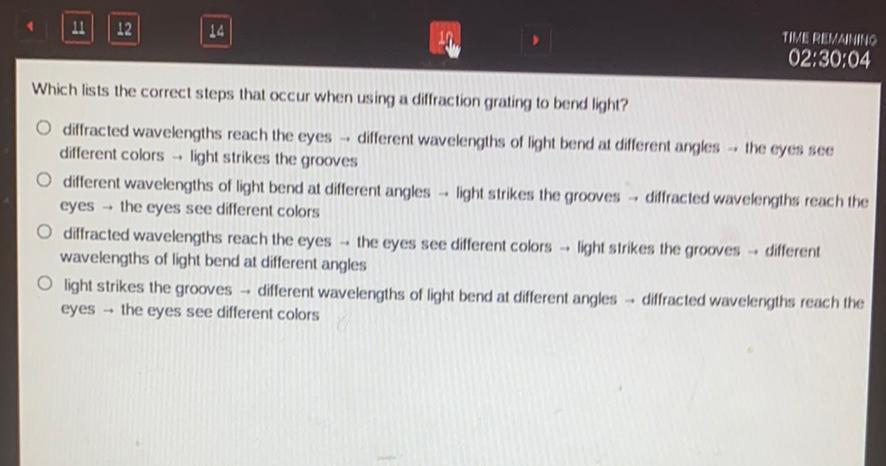Question:

# Which lists the correct steps that occur when using a diffraction grating to bend light? a. diffracted wavelengths reach the eyes → different wavelengths of light bend at different angles → the eyesWhich lists the correct steps that occur when using a diffraction grating to bend light? a. diffracted wavelengths reach the eyes → different wavelengths of light bend at different angles → the eyes see different colors → light strikes the grooves b. different wavelengths of light bend at different angles → light strikes the grooves → diffracted wavelengths reach the eyes→ the eyes see different colors c. diffracted wavelengths reach the eyes → the eyes see different colors → light strikes the grooves → different wavelengths of light bend at different angles d. light strikes the grooves → different wavelengths of light bend at different angles → diffracted wavelengths reach the eyes→ the eyes see different colors